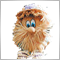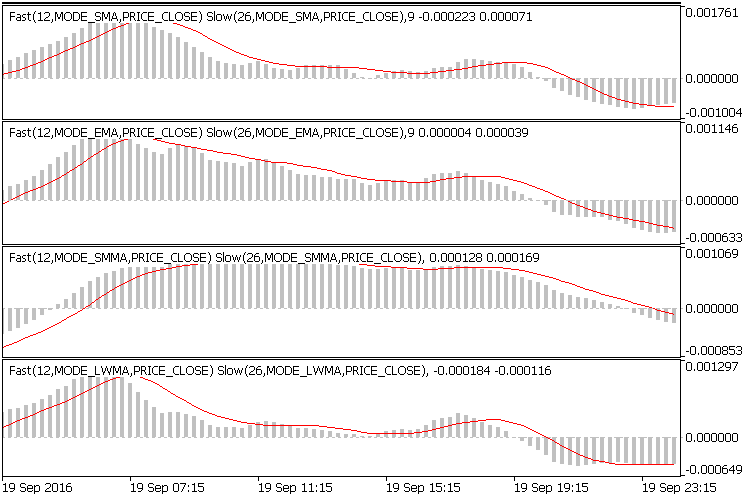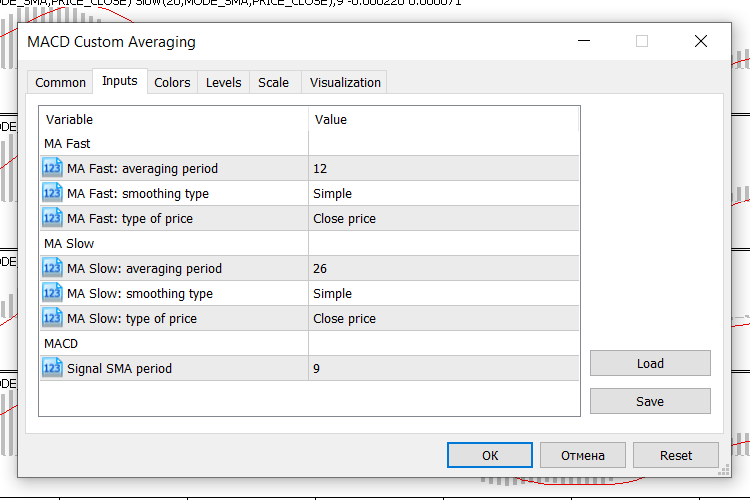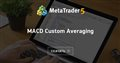# Modified MACD for MT5 inf problem275

Hello everybody!

There is this 3-10 Oscillator for MT4 which works like this:
```   for(int i=0; i<limit; i++)
ThreeTenBuffer[i]=iMA(NULL,0,FastSMA,0,MODE_SMA,PRICE_CLOSE,i)-iMA(NULL,0,SlowSMA,0,MODE_SMA,PRICE_CLOSE,i);
//---- signal line counted in the 2nd buffer
for(i=0; i<limit; i++)
SignalBuffer[i]=iMAOnArray(ThreeTenBuffer,Bars,SignalSMA,0,MODE_SMA,i);```
It uses the MODE_SMA for Fast & Slow MA's instead of the MODE_EMA in the standard MACD indicator.

Theoretically, it should be simple to modify the MACD.mq5 in the same mode.
I used the following code:
```//+------------------------------------------------------------------+
//|                                                         MACD.mq5 |
//|                        Copyright 2009, MetaQuotes Software Corp. |
//|                                              http://www.mql5.com |
//+------------------------------------------------------------------+
#property description "Moving Average Convergence/Divergence"
#include <MovingAverages.mqh>
//--- indicator settings
#property indicator_separate_window
#property indicator_buffers 4
#property indicator_plots   2
#property indicator_type1   DRAW_HISTOGRAM
#property indicator_type2   DRAW_LINE
#property indicator_color1  Silver
#property indicator_color2  Red
#property indicator_width1  2
#property indicator_width2  1
#property indicator_label1  "MACD"
#property indicator_label2  "Signal"
//--- input parameters
input int                InpFastEMA=12;               // Fast EMA period
input int                InpSlowEMA=26;               // Slow EMA period
input int                InpSignalSMA=9;              // Signal SMA period
input ENUM_APPLIED_PRICE InpAppliedPrice=PRICE_CLOSE; // Applied price
//--- indicator buffers
double                   ExtMacdBuffer[];
double                   ExtSignalBuffer[];
double                   ExtFastMaBuffer[];
double                   ExtSlowMaBuffer[];
//--- MA handles
int                      ExtFastMaHandle;
int                      ExtSlowMaHandle;
//+------------------------------------------------------------------+
//| Custom indicator initialization function                         |
//+------------------------------------------------------------------+
void OnInit()
{
//--- indicator buffers mapping
SetIndexBuffer(0,ExtMacdBuffer,INDICATOR_DATA);
SetIndexBuffer(1,ExtSignalBuffer,INDICATOR_DATA);
SetIndexBuffer(2,ExtFastMaBuffer,INDICATOR_CALCULATIONS);
SetIndexBuffer(3,ExtSlowMaBuffer,INDICATOR_CALCULATIONS);
//--- sets first bar from what index will be drawn
PlotIndexSetInteger(1,PLOT_DRAW_BEGIN,InpSignalSMA-1);
//--- name for Dindicator subwindow label
IndicatorSetString(INDICATOR_SHORTNAME,"MACD("+string(InpFastEMA)+","+string(InpSlowEMA)+","+string(InpSignalSMA)+")");
//--- get MA handles
ExtFastMaHandle=iMA(NULL,0,InpFastEMA,0,MODE_EMA,InpAppliedPrice);
ExtSlowMaHandle=iMA(NULL,0,InpSlowEMA,0,MODE_EMA,InpAppliedPrice);
//--- initialization done
}
//+------------------------------------------------------------------+
//| Moving Averages Convergence/Divergence                           |
//+------------------------------------------------------------------+
int OnCalculate(const int rates_total,const int prev_calculated,
const datetime &Time[],
const double &Open[],
const double &High[],
const double &Low[],
const double &Close[],
const long &TickVolume[],
const long &Volume[],
{
//--- check for data
if(rates_total<InpSignalSMA)
return(0);
//--- not all data may be calculated
int calculated=BarsCalculated(ExtFastMaHandle);
if(calculated<rates_total)
{
Print("Not all data of ExtFastMaHandle is calculated (",calculated,"bars ). Error",GetLastError());
return(0);
}
calculated=BarsCalculated(ExtSlowMaHandle);
if(calculated<rates_total)
{
Print("Not all data of ExtSlowMaHandle is calculated (",calculated,"bars ). Error",GetLastError());
return(0);
}
//--- we can copy not all data
int to_copy;
if(prev_calculated>rates_total || prev_calculated<0) to_copy=rates_total;
else
{
to_copy=rates_total-prev_calculated;
if(prev_calculated>0) to_copy++;
}
//--- get Fast EMA buffer
if(IsStopped()) return(0); //Checking for stop flag
if(CopyBuffer(ExtFastMaHandle,0,0,to_copy,ExtFastMaBuffer)<=0)
{
Print("Getting fast EMA is failed! Error",GetLastError());
return(0);
}
//--- get SlowSMA buffer
if(IsStopped()) return(0); //Checking for stop flag
if(CopyBuffer(ExtSlowMaHandle,0,0,to_copy,ExtSlowMaBuffer)<=0)
{
Print("Getting slow SMA is failed! Error",GetLastError());
return(0);
}
//---
int limit;
if(prev_calculated==0)
limit=0;
else limit=prev_calculated-1;
//--- calculate MACD
for(int i=limit;i<rates_total && !IsStopped();i++)
{
ExtMacdBuffer[i]=ExtFastMaBuffer[i]-ExtSlowMaBuffer[i];
}
//--- calculate Signal
SimpleMAOnBuffer(rates_total,prev_calculated,0,InpSignalSMA,ExtMacdBuffer,ExtSignalBuffer);
//--- OnCalculate done. Return new prev_calculated.
return(rates_total);
}
//+------------------------------------------------------------------+
```
Thus, I do this:
```//--- get MA handles
ExtFastMaHandle=iMA(NULL,0,InpFastEMA,0,MODE_SMA,InpAppliedPrice);
ExtSlowMaHandle=iMA(NULL,0,InpSlowEMA,0,MODE_SMA,InpAppliedPrice);```
And the indicator stops working!
I get a blank picture, as in the atttached image, with "inf" & "-inf".
I wasn't able to get around this problem.
Files:Moderator
273996

All indicators are supplied with the terminal and indicators are supplied in open source code. MACD lies here: [data folder] \ MQL5 \ Indicators \ Examples \ MACD.mq5275

All indicators are supplied with the terminal and indicators are supplied in open source code. MACD lies here: [data folder] \ MQL5 \ Indicators \ Examples \ MACD.mq5

It was useless.
I used the one you suggested and got exactly the same result, meaning nothing.
Exactly the same result as described by me, above.
The indicator in the Examples is exactly the same one I used.
It only works in the MODE_EMA for the MA handles.
In any other of the available MA_METHOD's it does not work.Moderator
273996

It was useless.
I used the one you suggested and got exactly the same result, meaning nothing.
Exactly the same result as described by me, above.
The indicator in the Examples is exactly the same one I used.
It only works in the MODE_EMA for the MA handles.
In any other of the available MA_METHOD's it does not work.

MACD Custom Averaging

### Indicator idea

Now in the MACD indicator for Moving Average 'Fast' and 'Slow' you can configure the averaging method and price type.Rice. 1. MACD Custom AveragingRice. 2. MACD Custom Averaging - parametersMACD Custom Averaging
• www.mql5.com
Модифицированный индикатор MACD: можно настаивать параметры обоих Moving Average275

MACD Custom Averaging

### Indicator idea

Now in the MACD indicator for Moving Average 'Fast' and 'Slow' you can configure the averaging method and price type.

Rice. 1. MACD Custom Averaging

Rice. 2. MACD Custom Averaging - parameters

Now thanking you very much!275